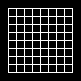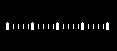# PgDrawGrid()

Draw a grid

## Synopsis:

```int PgDrawGrid( PhRect_t const *r,
PhPoint_t const *g );```

## Description:

This function fills the rectangle specified by r with the number of divisions specified by g. The number of lines drawn equals the number of divisions plus 1.

This function builds a draw command to draw the grid. The size of the grid is defined by the r argument with g.x+1 vertical lines and g.y+1 horizontal lines. If g.x is 0, no vertical lines are drawn; if g.y is 0, no horizontal lines are drawn.

## Returns:

0
Success.
-1
The draw buffer is too small to hold the current draw state and the draw command.

## Examples:

The following example uses PgDrawGrid() to make a grid of 8 squares by 8 squares; each square is 8 by 8 pixels:

```void GridStandard() {
PhRect_t r = { 8, 8, 72, 72 };
PhPoint_t g = { 8, 8 };

PgSetStrokeColor( Pg_WHITE );
PgDrawGrid( &r, &g );
}```

This code draws:The following example uses PgDrawGrid() to generate 20 ticks. Every 5th tick is made larger by calling PgDrawGrid() again with different parameters:

```void GridTicks() {
PhRect_t r = { 8, 24, 108, 28 };
PhPoint_t g = { 20, 0 };

PgSetStrokeColor( Pg_WHITE );
PgDrawGrid( &r, &g );
r.ul.y-=1;
r.lr.y+=1;
g.x=4;
PgSetStrokeWidth( 3 );
PgSetStrokeCap( Pg_POINT_CAP );
PgDrawGrid( &r, &g );
PgSetStrokeWidth( 0 );
}```

This code draws:## Classification:

Photon

Safety:
Interrupt handler No
Signal handler No
Thread No